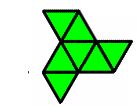Distances

Prepared by:

Joseph Malkevitch
Department of Mathematics and Computer Studies
York College (CUNY)
Jamaica, New York

email:

malkevitch@york.cuny.edu

web page:

http://york.cuny.edu/~malk

There are only three tilings of the Euclidean plane by regular convex polygons. These are the tilings by triangles, 6 at a vertex, by squares, 4 at a vertex, and by hexagons, 3 at a vertex.

If one has a finite connected clump of one of these tilings the resulting clusters of polygons are referred to as polyiamonds, polyominoes and polyhexes. Samples of polyiamonds, polyominoes, and polyhexes are shown in Figures 1-3, respectively. I will assume all of the edges in the diagrams have unit length.Figure 1 (Polyiamond, Courtesy of Wiki)Figure 2 (Polyominoe, Courtesy of Wiki)Figure 3 (Polyhex, Courtesy of Wiki)

A member of one of these collections can be parameterized in various ways:

a. Number of (finite) faces (considered as a graph drawn in the plane)

b. Length of the boundary (number of sides of the infinite face)

c. Area of the region in the Euclidean plane

d. Number of points (vertices considered as graphs)

A line of interesting research in combinatorics and geometry, inspired in part by questions of Paul Erdös, deal with distance questions related to points in the plane (or in spaces of higher dimension). The general plane settings, though some of these questions have already been well studied (or are not very interesting), are given below.

a. Given a collection of n points in the plane:

i. Find the smallest and largest number of distinct distances (Euclidean distance) possible.

ii. Find the smallest and largest number of unit distances possible.

One can think of the points as being given or one can think of putting the points where one wants them so as to achieve a particular goal with regard to the distances involved.

b. Given a collection of n points in the plane, where we have r distinct
distances, r < n . How small can r get?

It is possible that "formulas" for numbers of distances in terms of a particular parameter might be found, but it is probably more realistic to find results that are asymptotic in a particular parameter.

While there are many results in recent years that have extended "classical" work on these types of problems, what has recently been looked at are questions of this kind, where the set of points involved are not "general" but have more structure.

In this spirit the questions below are raised.

Problem 1

Investigate distance problems for points which are vertices of:

a. a polyiamond

b. a polyominoe

c. a polyhex

Problem 2

Restricted to polyiamonds, polyomines, and polyhexes comment, compare, and contrast the results of distance questions.

Problem 3

Extend problems of this kind to Archimedean tilings of the plane.

Reference:

Garibaldi, J. and A. Iosevich, S. Senger, The Erdös Distance Problem, American Mathematical Society, Providence, 2011.